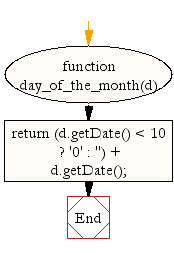# JavaScript: Get the day of the month, 2 digits with leading zeros

## JavaScript Datetime: Exercise-19 with Solution

Write a JavaScript function to get the day of the month, 2 digits with leading zeros.

Test Data :
d= new Date(2015, 10, 1);
console.log(day_of_the_month(d));
19
01

Sample Solution:-

HTML Code:

``````<!DOCTYPE html>
<html>
<meta charset="utf-8">
<title>JavaScript function to get the day of the month, 2 digits with leading zeros.</title>
<body>

</body>
</html>
```
```

JavaScript Code:

``````function day_of_the_month(d)
{
return (d.getDate() < 10 ? '0' : '') + d.getDate();
}

d= new Date();
console.log(day_of_the_month(d));

d= new Date(2015, 10, 1);
console.log(day_of_the_month(d));
```
```

Sample Output:

```19
01
```

Flowchart:Live Demo:

See the Pen JavaScript - Get the day of the month, 2 digits with leading zeros-date-ex-19 by w3resource (@w3resource) on CodePen.

Improve this sample solution and post your code through Disqus

What is the difficulty level of this exercise?

﻿

## JavaScript: Tips of the Day

|| operator

```const one = false || {} || null;
const two = null || false || '';
const three = [] || 0 || true;

console.log(one, two, three);
```

With the || operator, we can return the first truthy operand. If all values are falsy, the last operand gets returned.
(false || {} || null): the empty object {} is a truthy value. This is the first (and only) truthy value, which gets returned. one is equal to {}.
(null || false || ""): all operands are falsy values. This means that the last operand, "" gets returned. two is equal to "".
([] || 0 || ""): the empty array[] is a truthy value. This is the first truthy value, which gets returned. three is equal to [].

Ref: https://bit.ly/3jFRBje